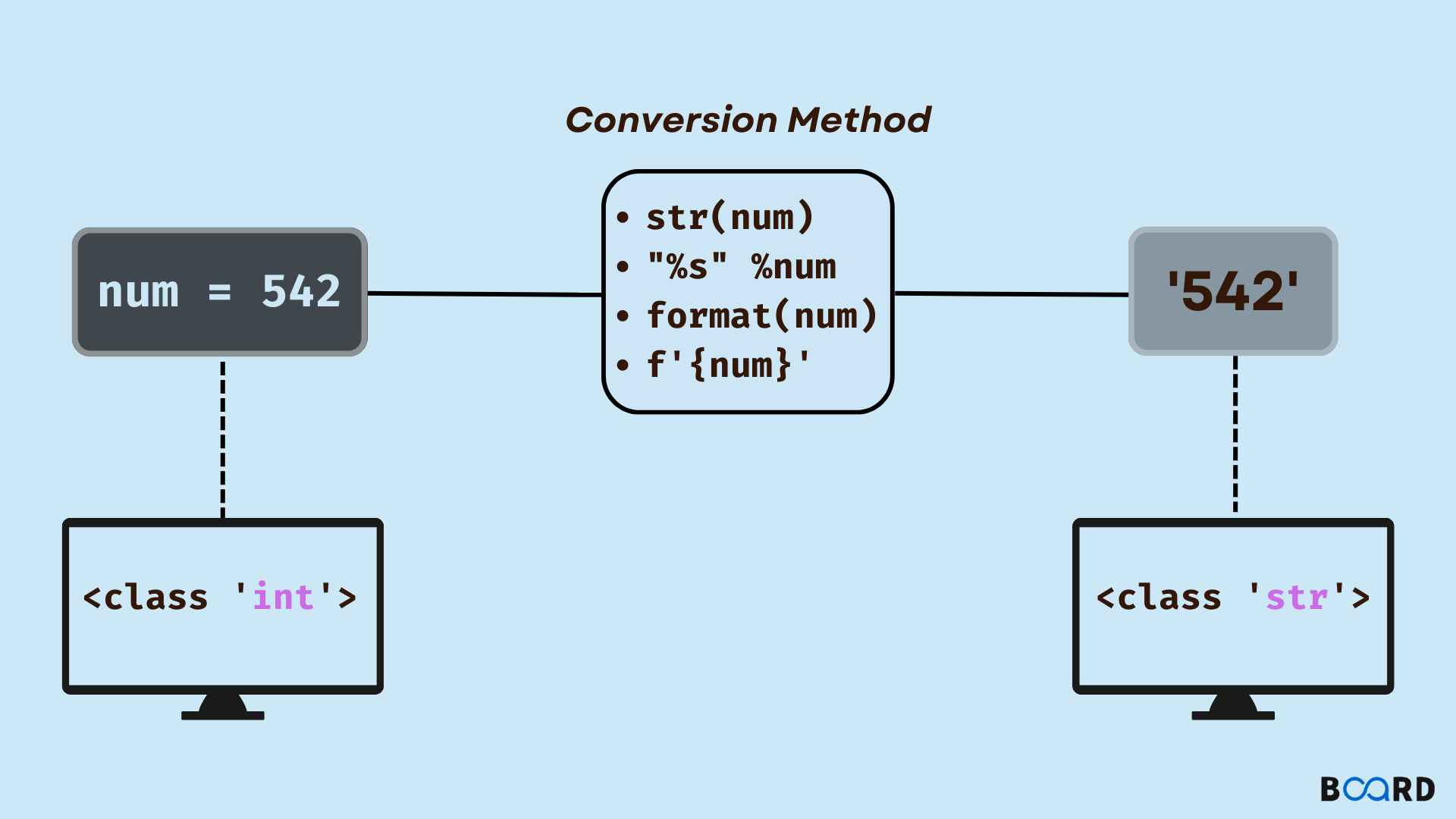Datatype conversion Python

# Int to String in Python: How?## Introduction

In Python programming, we might run into a number of issues that call for the use of an integer data type as a string data type. To perform string specific operations on numbers, first they must be converted to their string counterparts by type-casting. A string can be created from an integer using a variety of techniques, including the str() function, the %s keyword, the format() function, and f-strings.

## Exploring Ways to Convert Int to String

Examples of conversions from int to string using various techniques:

### Using str() function in Python

The str() method in Python uses typecasting to convert any Python data type into a string representation of the original object. The object's string representation is the str() function's return value. Consequently, a string may be created from an integer by using the str() method. Let's see a str() function example that turns an int into a string (typecasting).

Code:

 num = 5print('Data type of "num" before conversion:', type(num))converted_num = str(num)print('Data type of "num" after conversion:', type(converted_num))

Output:

 Data type of "num" before conversion: Data type of "num" after conversion:
write your code here: Coding Playground

Explanation:

In the aforementioned example, we first initialize the integer "num," and when we verify its data type, the result indicates that it is a "int." The str() method is then used to transform the value into a string. The output shows the outcome, which is that the converted data type is "str."

### Using %s keyword in Python

Code:

 num = 5print('Data type of "num" before conversion:', type(num))converted_num = "% s" % numprint('Data type of "num" after conversion:', type(converted_num))

Output:

 Data type of "num" before conversion: Data type of "num" after conversion:
write your code here: Coding Playground

Explanation:

When we verify the data type of "num" in the example above, it is shown that the data type is "int." The Python %s keyword is then used to turn the value into a string. The output shows the outcome, which is that the converted data type is "str."

### Using format() function in Python

Code:

 num = 5print('Data type of "num" before conversion:', type(num))converted_num = "{}" format(num)print('Data type of "num" after conversion:', type(converted_num))

Output:

 Data type of "num" before conversion: Data type of "num" after conversion:
write your code here: Coding Playground

Explanation:

When we verify the data type of "num" in the example above, it is shown that the data type is "int." The Python format() method is then used to turn the value into a string. The output shows the outcome, which is that the converted data type is "str."

### Using f-strings in Python

Code:

 num = 5print('Data type of "num" before conversion:', type(num))converted_num = f'{num}'print('Data type of "num" after conversion:', type(converted_num))

Output:

 Data type of "num" before conversion: Data type of "num" after conversion:
write your code here: Coding Playground

Explanation:

When we verify the data type of "num" in the example above, it is shown that the data type is "int." Then, we use Python's f-strings to turn the value into a string. The output shows the outcome, which is that the converted data type is "str."

## Conclusion

• The str() function, the %s keyword, the format() function, and the f-strings method are all options for type casting an integer into a string.
• The representation of values may also be formatted while being converted into a string using the format() function and the f-strings method.
• Despite the fact that there are four different ways to typecast an integer into a string, the str() function is the most useful one.